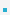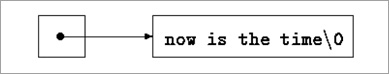5. Strings as Arrays

5.1 StringsA string constant, written as "I am a string“ is an array of characters.Strings stored as null-terminated character arrays (last character == ‘\0’)

 char str[] = “This is a string.”; char *pc = str; *(pc+10) = ‘S’; puts(str); // prints “This is a String.”There is an important difference between the following definitions:

 char amessage[] = “now is the time”; // an array char *pmessage = “now is the time”; // a pointer

– amessage is an array, just big enough to hold the sequence of characters and '\0' that initializes it.– pmessage is a pointer, initialized to point to a string constant.5.2 String Utility FunctionsString functions in standard header string.h

 Type Functions Meaning Copy char *strcpy(strto, strfrom) copy strfrom to strto char *strncpy(strto, strfrom, n) copy n chars from strfrom to strto Comparison int strcmp(str1, str2) compare str1, str2; return 0 if equal, positive if str1>str2, negative if str1

Example: strcpy()

 /* strcpy: copy from to to */ * strcpy: array subscript version */ void strcpy(char *to, char *from) {      int i;      i = 0;      while ((to[i] = from[i]) != ‘\0’)           i++; } /* strcpy: pointer version */ void strcpy(char *to, char *from) {      int i;      i = 0;      while ((*to = *from) != ‘\0’) {           to++;           from++;      } } /* strcpy: pointer version 2 */ void strcpy(char *to, char *from) { while ((*to++ = *from++) != ‘\0’) ; } /* strcpy: pointer version 3 */ void strcpy(char *to, char *from) {      while (*to++ = *from++)           ; }

Example: strcmp()

 /* strcmp: return <0 if s0 if s>t */ /* strcmp: array version */ int strcmp(char *s, char *t) {     int i;     for (i=0; s[i]==t[i]; i++)         if (s[i]==‘\0’)            return 0;     return s[i]-t[i]; } /* strcmp: pointer version */ int strcmp(char *s, char *t) {     for (; *s==*t; s++, t++)          if (*s==‘\0’)             return 0;     return *s-*t; }

 이전페이지 / 6 / 다음페이지